# Calculus 1 : Local Minimum

## Example Questions

1 3 Next →

### Example Question #51 : Graphing Functions

Find the local minimum of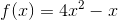.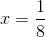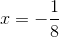Explanation:

First, take the derivative of the function, remembering to multiply the exponent by the coefficeint and then subtracting one from the exponent. Therefore, the derivative is: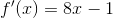. Then, set it equal to 0 to get your critical point: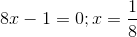. Then, test a value on either side of your critical point and plug into the derivative. To the left, the value is negative. To the right, the value is positive. Therefore, your local minimum is at.

### Example Question #52 : Graphing Functions

Given the function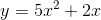, find its local minimum.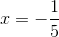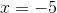Explanation:

To find the local minimum of a function, one must find the critical points (where the derivative is equal to zero).

Taking the derivative of the function above using the power rule,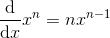.

The derivative is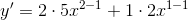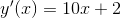.

Setting this equal to zero and solving for x gets you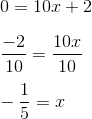To see if this indeed is a local minimum one must plug in values below and above this value of x to see if the derivative is positive (increasing) or negative (decreasing) around that point.

If you plug -2 into the equation for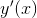you get -18 (decreasing), and plugging in 0, you get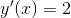(increasing).

Thus,is a local minimum.

1 3 Next →

### All Calculus 1 Resources